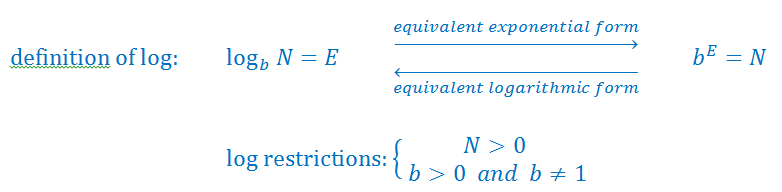# Converting from logarithmic form to exponential form

## What is exponential form in math?

The log form and exponential form are actually inverses of each other. Exponentials happen when a number is raised to a certain power. It is a shorter way to show that a number is repeatedly multiplied a number of times by itself. An example of an exponential form number would be that in order to show 3x3x3x3, we'd instead write 34. The exponent is the little number 4, and it shows how many times the number is to be multiplied by itself. The base is 3, and it is what is being multiplied.

A logarithm of a number, on the other hand, is the exponent that a base has to be raised to make that number.

You probably recall how to change exponential form to radical form. But in this chapter, we're looking at something a little different. How do we convert log form to exponential form and vice versa? What's does converting log vs exponential form ultimately look like? Let's explore this through an example.

Question: Convert $lo{g_2}\;8\; = 3$into exponential form.

Solution:

$lo{g_2}\;8\; = 3$

Convert into this form: ${b^E} = N$

${2^3} = 8$

How does this work? Let's take a step-by-step look into how we change the form of the equation. The relationship between log and exponential form makes converting between the two easy. ${b^E} = N$ is equalled to $lo{g_b}\left( y \right)\; = x$ is the relationship that we're referring to. What exactly does the letters in ${b^E} = N$mean?

The lower case b stands for the "base", which is equaled to the small number beside log. N is the "number", which corresponds to the big number beside log. Lastly, we've got the "E", which stands for "exponent" and it is what the log expression is equal to. Notice that the base in both the log and exponential form of the equation is "b", but it is mainly the x and y in the relationship that switches sides. Whatever the log form equation equaled becomes the exponent, and vice versa.

Using the relationship, we can easily see how by looking at a log form equation, we can convert it to exponential form. Simply by moving the corresponding parts of the log form equations into ${b^E} = N$ format, you can find the exponential form of log. To recap: In order to change a logarithmic form function to an exponential one, first find the base, which is the little number next to the word "log". The base doesn't change sides, but the other numbers (the exponent and the "number") do. Lastly, remove the word "log" from the equation and you'll have successfully converted the equation!

You can also use the same idea to convert exponential form to logarithmic by doing the reverse of what we explored in this article. Continue trying out our practice problems to see the relationship between log and exponentials used in more advanced situations to make sure you can convert any equation in your upcoming tests! Here are also some additional walkthroughs to demonstrate the concept.

### Converting from logarithmic form to exponential form

#### Lessons

• Introduction• 1.
Rewriting the Equations in Exponential Form
Convert from log form to exponential form
a)
$\log_28 = 3$

b)
123 = 4$\log_b5$

• 2.
Solving Equations by Converting From Logarithmic to Exponential Form
Solve for x
a)
$\log_3x = 4$

b)
$\log_525 = x$

c)
given: $\log_9w = 0.5$ and $\log_2x = w$

• 3.
Solve for ${y}$:
$\log_5( \frac{y}{2}) = x$

• 4.
A Logarithmic Expression Inside Another Logarithmic Expression
Solve for ${x}$:
$\log_8( \log_7(5-x)) = \frac{1}{3}$

• 5.
Extension: Solving Equations by Converting From Logarithmic to Exponential Form
a)
solve for ${ y: }$
$\log_{10}0.00001 = y$

b)
solve for ${ x: }$
3 = 5$\log_x8$

c)
solve for ${ y: }$
$\log_3(5y-30)=2x+20\$